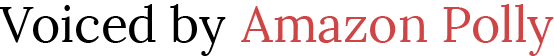## Machine Learning

### Innovate much?

“AI has no pretensions whatever to originate anything. It can do whatever we know how to order it to perform. It can follow analysis; but it has no power of anticipating any analytical relations or truths. Its province is to assist us to making available what we are already acquainted with.”

August Ada King, Countess of Lovelace, 1842

We use machine learning and time-series forecasting to scale in the following domains:

RETAIL – For retail demand forecasting.

INVENTORY PLANNING – For supply chain and inventory planning

WORK FORCE – For work force planning

WEB TRAFFIC – For estimating future web traffic

METRICS – For forecasting metrics, such as revenue and cash flow

CUSTOM – For all other types of time-series forecasting

#### Forecasting Models

CNN-QR – CNN-QR, Convolutional Neural Network – Quantile Regression, is a proprietary machine learning algorithm for forecasting time series using causal convolutional neural networks (CNNs). CNN-QR works best with large datasets containing hundreds of time series. It accepts item metadata, and is the only Forecast algorithm that accepts related time series data without future values.

DeepAR+ – DeepAR+ is a proprietary machine learning algorithm for forecasting time series using recurrent neural networks (RNNs). DeepAR+ works best with large datasets containing hundreds of feature time series. The algorithm accepts forward-looking related time series and item metadata.

Prophet – Prophet is a time series forecasting algorithm based on an additive model where non-linear trends are fit with yearly, weekly, and daily seasonality. It works best with time series with strong seasonal effects and several seasons of historical data.

NPTS – Non-Parametric Time Series (NPTS) proprietary algorithm is a scalable, probabilistic baseline forecaster. NPTS is especially useful when working with sparse or intermittent time series. Forecast provides four algorithm variants: Standard NPTS, Seasonal NPTS, Climatological Forecaster, and Seasonal Climatological Forecaster.

ARIMA – Autoregressive Integrated Moving Average (ARIMA) is a commonly used statistical algorithm for time-series forecasting. The algorithm is especially useful for simple datasets with under 100 time series.

ETS – Exponential Smoothing (ETS) is a commonly used statistical algorithm for time-series forecasting. The algorithm is especially useful for simple datasets with under 100 time series, and datasets with seasonality patterns. ETS computes a weighted average over all observations in the time series dataset as its prediction, with exponentially decreasing weights over time.

#### Statistics Metrics

MAPE – Mean Absolute Percentage Error (MAPE) takes the absolute value of the percentage error between observed and predicted values for each unit of time, then averages those values. A lower value indicates a more accurate model.

MASE – Mean Absolute Scaled Error (MASE) is calculated by dividing the average error by a scaling factor. This scaling factor is dependent on the seasonality value, m, which is selected based on the forecast frequency. A lower value indicates a more accurate model.

RMSE– Root Mean Square Error (RMSE) is the square root of the average of squared errors, and is therefore more sensitive to outliers than other accuracy metrics. A lower value indicates a more accurate model. Forecast now uses the mean forecast.

WAPE – The Weighted Absolute Percentage Error (WAPE) measures the overall deviation of forecasted values from observed values. WAPE is calculated by taking the sum of observed values and the sum of predicted values, and calculating the error between those two values. A lower value indicates a more accurate model.

 Listen to post